# Velocity and Acceleration Algebra

Here we will take a look at the equation that allows us to solve for the final velocity when the object is constantly accelerating. That equation is: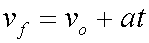Final velocity equals original velocity plus acceleration times time.

We will use algebra to solve the above equation for each of its variables.

The equation in its original form is already solved for final velocity, vf:Here, we will solve for original velocity, vo:Begin here.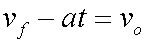Subtract at from each side.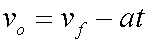Rearrange by switching left and right sides.

Now, let's see the equation solved for acceleration, a:Start here.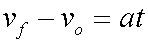Subtract vo from each side.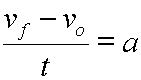Divide each side by t.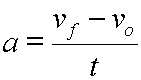Rearrange by switching left and right sides.(Basically, this equation is the definition of acceleration.)

Now, let's see the equation solved for time, t:Begin here.Subtract vo from each side.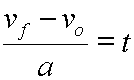Divide both sides by a.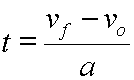Rearrange by switching left and right sides.

Custom Search